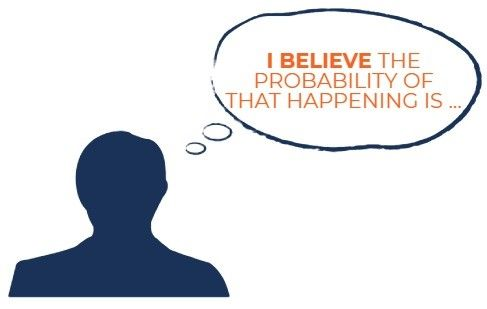# Subjective Probability

The probability of something happening based on an individual’s own experience or personal judgment

## What is Subjective Probability?

Subjective probability refers to the probability of something happening based on an individual’s own experience or personal judgment. A subjective probability is not based on market data or historical information and differs from person to person. In other words, it is created from the opinion of an individual and is not based on fact.### Understanding Subjective Probability

In most types of probability, quantitative information is interpreted to determine the likelihood of something happening. However, subjective probability does not base its probability on quantitative information, is affected by personal beliefs, and contains no formal calculations.

For example, an individual who believes that there is a 70% chance that the S&P 500 will increase tomorrow is using subjective probability. Another individual asked the same question would likely offer a different probability of the S&P 500 increasing tomorrow – it is due to the different personal beliefs held by different individuals.

When the probability of something happening differs from person to person, it is likely a subjective probability.

### Examples of Subjective Probability

#### Example 1

An analyst is asked the probability of the S&P 500 will hit all-time highs in the coming months. The analyst looks at past trends and current market conditions and estimates that the probability of the S&P 500 will hit all-time highs at 20%.

#### Example 2

An individual is asked the probability of a dice roll yielding a 6. The individual looks at the past three rolls and notes that 6 came up in all instances. The individual believes that the probability of the next dice roll yielding a 6 is at 30%. Although the mathematical prediction is incorrect (the probability is 16.67%), the individual’s personal experience of the dice roll yielding 6 in three instances created a situation where he used subjective probability.

### Subjective Probability in the News: Probability of a U.S. Recession?

On June 30, 2019, the New York Fed’s recession probability model warned that there was a 30% probability of a recession within the next 12 months (from June 30, 2019 to June 30, 2020). If other economists are asked regarding the probability of a recession within the next 12 months, they would likely provide a different estimate. The probability of a recession is based on opinion – not fact – and is, therefore, an example of a subjective probability.

### Other Types of Probabilities

Apart from subjective probabilities, there are two other main types of probabilities:

#### 1. Empirical probability

Empirical probability refers to a probability that is based on historical data. For example, if three coin tosses yielded a head, the empirical probability of getting a head in a coin toss is 100%.

#### 2. Classical probability

Classical probability refers to a probability that is based on formal reasoning. For example, the classical probability of getting a head in a coin toss is 50%.

Subjective probability is the only type of probability that incorporates personal beliefs. Empirical and classical probabilities are objective probabilities.

### More Resources

CFI is the official provider of the global Financial Modeling & Valuation Analyst (FMVA)™ certification program, designed to help anyone become a world-class financial analyst. To keep advancing your career, the additional resources below will be useful:

• Basic Statistics Concepts in Finance
• Binomial Distribution
• Conditional Probability
• Independent Events

### Financial Analyst Certification

Become a certified Financial Modeling and Valuation Analyst (FMVA)® by completing CFI’s online financial modeling classes and training program!